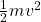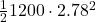## Calculate the Kinetic Energy when the mass of the car is 1,200kg and the speed is 2.78 m/s. ​

Question

Calculate the Kinetic Energy when the mass of the car is 1,200kg and the speed
is 2.78 m/s. ​

in progress 0
2 months 2021-08-01T11:24:44+00:00 1 Answers 1 views 0

Kinetic energy of the car = 4637.04 J

Explanation:

Mass of the car (m) =1200 kg

speed of the car (v) = 2.78 m/sec

Kinetic energy =Putting the values of m and v,

Hence, Kinetic Energy of the car ==  4637.04 J